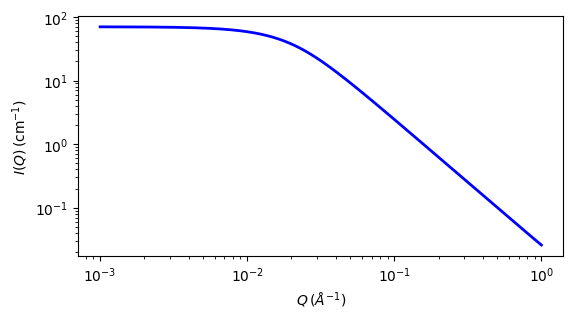# mono_gauss_coil

Scattering from monodisperse polymer coils

Parameter

Description

Units

Default value

scale

Scale factor or Volume fraction

None

1

background

Source background

cm-1

0.001

i_zero

Intensity at q=0

cm-1

70

rg

75

The returned value is scaled to units of cm-1 sr-1, absolute scale.

This Debye Gaussian coil model strictly describes the scattering from monodisperse polymer chains in theta solvents or polymer melts, conditions under which the distances between segments follow a Gaussian distribution. Provided the number of segments is large (ie, high molecular weight polymers) the single-chain form factor P(Q) is that described by Debye (1947).

To describe the scattering from polydisperse polymer chains see the poly_gauss_coil model.

Definition

$I(q) = \text{scale} \cdot I_0 \cdot P(q) + \text{background}$

where

$\begin{split}I_0 &= \phi_\text{poly} \cdot V \cdot (\rho_\text{poly} - \rho_\text{solv})^2 \\ P(q) &= 2 [\exp(-Z) + Z - 1] / Z^2 \\ Z &= (q R_g)^2 \\ V &= M / (N_A \delta)\end{split}$

Here, $$\phi_\text{poly}$$ is the volume fraction of polymer, $$V$$ is the volume of a polymer coil, M is the molecular weight of the polymer, $$N_A$$ is Avogadro’s Number, $$\delta$$ is the bulk density of the polymer, $$\rho_\text{poly}$$ is the sld of the polymer, $$\rho\text{solv}$$ is the sld of the solvent, and $$R_g$$ is the radius of gyration of the polymer coil.

The 2D scattering intensity is calculated in the same way as the 1D, but where the q vector is redefined as

$q = \sqrt{q_x^2 + q_y^2}$Fig. 112 1D plot corresponding to the default parameters of the model.

Source

References

1. P Debye, J. Phys. Colloid. Chem., 51 (1947) 18.

2. R J Roe, Methods of X-Ray and Neutron Scattering in Polymer Science, Oxford University Press, New York (2000).

3. http://www.ncnr.nist.gov/staff/hammouda/distance_learning/chapter_28.pdf

Authorship and Verification

• Author: